### What is Intelligence: Deep Learning

DEEP LEARNING

The power of deep learning is in its ability to generalize patterns, in its most common application in image recognition, it takes an image and generalizes away the details of the image in a lossy way to arrive at the most abstract representation of that image such that when an image that possesses the same features as the one being generalized is presented to it, it recognizes it as the same image because it possesses the same features. This is very powerful and it is close to the way humans actually perform pattern recognition.

At its most fundamental the technique is very simple. Input data are represented as a vector of numbers, a vector of numbers can be viewed simply as a list of numbers, and the output is also a vector of numbers which represent probabilities.

This is a very simple scenario of deep learning that I seek to use as an aid to guide our understanding. There are a lot of complex variations of how the input and output should look like. In another scenario, the output is not a vector but a scalar. A scalar is simply a single number! I am using the technical terms and providing explanations so that you will know what they mean if you see them elsewhere if you are not already very versed in machine learning.

So in the scenario where we have a vector of numbers as input and a vector of numbers as output, the goal of a deep learning system is to “learn” the output vector. In a classification system, these vector of probabilities will be simplified to a situation where we choose the highest probability to represent what class the input vector represents. Remember that classification is simply boxing different items in different categories.

What makes machine learning sometimes difficult to understand is that it requires a certain alteration of our basic thinking process. Most of the times we are used to thinking of things as moving from some starting point to some endpoint or goal. But in machine learning, we have the starting point or input data and the ending point, output data, and our goal is to “learn”, that is finding out a stable connection between the input and the output data. This connection is given as a collection of “weights”.

The whole algorithmic system of deep learning involves discovering the appropriate set of weights that represents the connection between certain input data and output data. We are concerned with the middle and not the end goal when we are doing machine learning. Deep learning is a specialization of machine learning.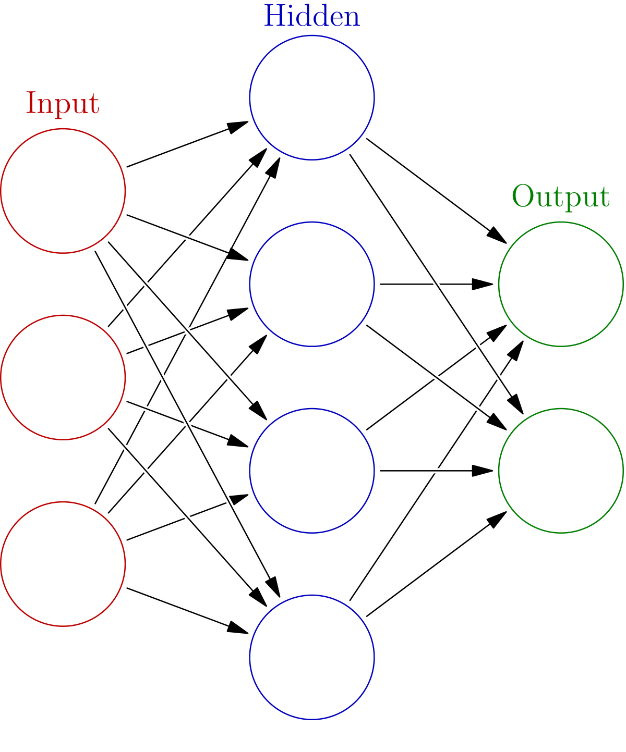Single Layer Neural Network

So why is it deep? It is deep because we cannot represent the extracted features of complicated input like the image of a cat, simple for humans but complicated for machines, with a single list of numbers. In machine learning, we are seeking to learn a generalized set of weights that can represent not just one cat image or one dog image or any other image, but we want weights that represent all possible cat images, that is why it is called generalizing.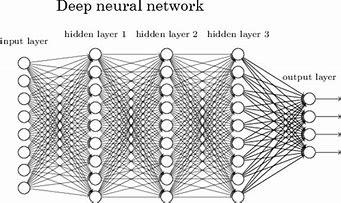If given a million cat images, of different breeds, colours, postures, etc., what is the most generalized “cat” we can come up with? This is the question we solve using deep learning techniques. So since we cannot get just one vector containing the most general representation of cats, we make the network deeper, meaning we create other layers for extracting information from the input layer. Each layer is also a vector containing weights. When we have successfully trained our network of layers we eventually arrive at a learned representation of what our input data, in this case, that of a cat.

These layers of weights or list of lists of vectors holding weights are usually called a weight matrix. So in finality deep learning enables us to learn a weight matrix of some data. This weight matrix is also called a representation of the input data. The power of the weight matrix is the fact that we could just add another layer at the tail end of the stacks of layers which contains a list of probabilities that summarises our data and tells us which item of which class we have supplied as input to the network.

I know you might be confused about this last layer because initially, I said that we are usually provided with a set of inputs and outputs and our goal is to learn the middle data which are the weights, now I am saying that we add a last layer to the mix to get probabilities. I am not pulling wool over your eyes, as you will see a little more explanation will clear the air.

Now during our training in a typical classification task, our goal is to arrive at a set of weights that best represent our input data, so we are given input and the desired output we seek to reach. Now during training how do we check that we have the desired output? We do this by comparing our original ideal output with the output that we have generated by the training system. If the difference between this original ideal output and that which our network has come up with during training is small then we have arrived at the best set of weights, that is the previous layers, which best represents our input data. If the difference is large, we might have to do more training till we reached a point where it becomes slow and difficult to improve the network’s output and when we have obtained suitable closeness to the ideal data. Training can be done with an algorithm called Stochastic Gradient Descent (SGD). This algorithm optimizes the cost function which is reducing the distance between the ideal output and that generated by the network. SGD uses backpropagation algorithm in the background to update the weight values in our network as we gradually hone on the best set of weights that best generalizes our input data.

This closeness is what we refer to as the accuracy of the training process. Our goal is to increase the accuracy of the training by reducing the difference between the ideal output and the output obtained from our training. The name for this difference between ideal and realized output in machine learning parlance is called the loss, or error. Loss is a quite counterintuitive name and sometimes I like using the term error because it is simple to explain. We can summarise the entire deep learning training as trying to reduce the error between our predicated output and the ideal output.

I have used the term probability without explaining much of what it is. The field of probability is an enormous field of mathematics and it is not my goal to teach you about that in this book. But I will give you a very practical understanding of what probability is. A probability is a number between 0 and 1 which represents the likelihood of an event to occur. In this system of representation, if we ascribe 0 (zero) probability to an event, we are simply saying that it is extremely unlikely for this event to occur. A probability of 1 will then mean that the event is highly likely to occur. All the numbers between 0 and 1, therefore, represent different degrees of likelihood of an event occurring. A probability of 0.5 means that an event is likely to occur or not occur with equal probability and 0.6 means that an event is more likely to occur than not occur. So you get the gist.

So what is this training process that we have been talking about? In neural networks, we train a network by adjusting the weights in the different layers of the network.

The process of training is divided into two stages, the feedforward stage and backpropagation stage. In the feedforward stage, we initialize our network with randomly chosen weights. Then we forward propagate these weights by performing a series of matrix multiplication of our randomly initialized weights with our input vector.

After the forward propagation phase, we compare the output of our network with the label of our ideal output using our loss function. After this we obtain some error, that is the difference between the desired output and the output we have obtained, and then we backpropagate these error updating the weights in the network. The goal of training a neural network is to find the best set of weights that generalizes the input data. We want weights such that when we multiply these weights with some input they produce the right probability that matches well with our targets.

The lines connecting one node (the circles in the images above) with other nodes in the graphs in the pictures above are called edges according to graph theory. The weight is a number that gives importance to each edge. An edge with a large weight is more important than an edge with a low weight. We can mentally visualize weights on the edges of the network as either representing the length or the thickness of the lines connecting the nodes. In the pictorial representation of DNNs (Deep Neural Networks) as we have above, for the economy of display we do not lengthen or thicken the lines connecting the nodes. But you can imagine that a bigger weight will result in a thicker or longer line in the graph that represents the neural network.

The nodes of a neural network, that is the circles in the pictures above, is what is referred to as a “neuron”, an idealization of a neuron in the brain of a biological entity.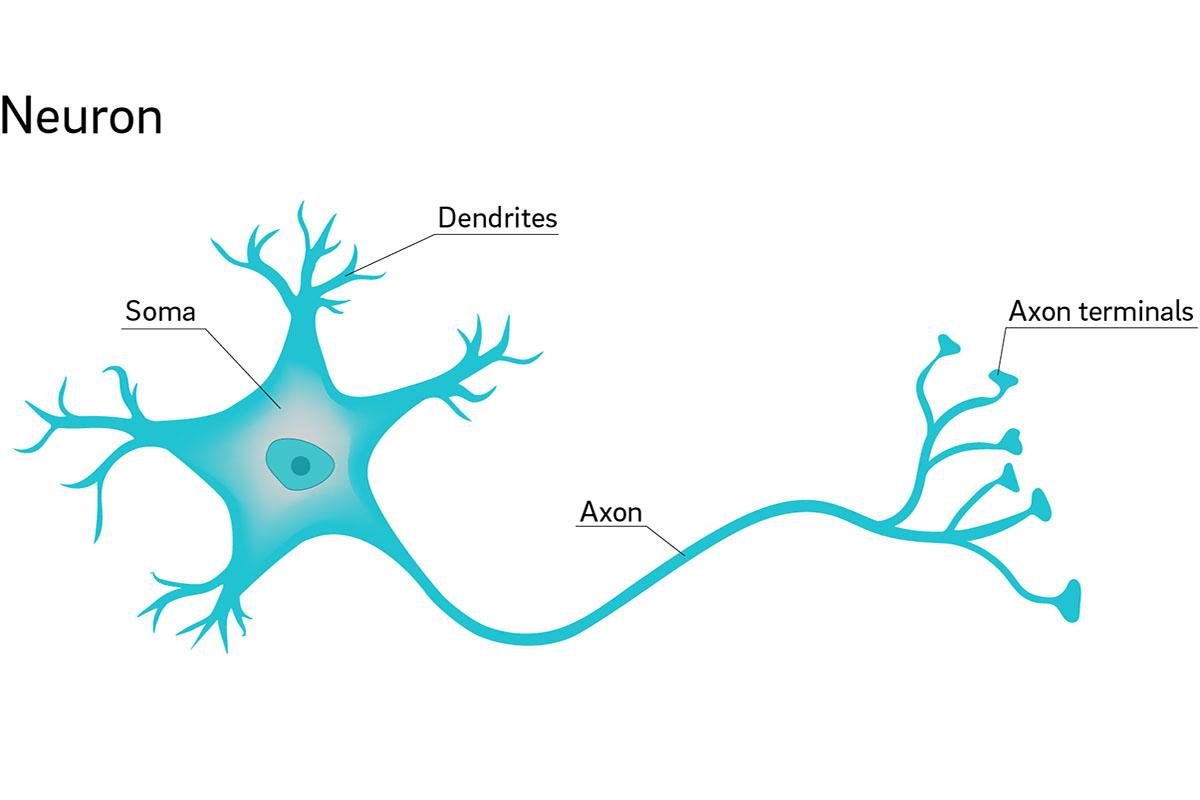Why is it called a neuron? Although we do not know for sure how the brain does what it does, and even if it is “calculating” anything in the usual sense of the word calculation. From studies of the brain, we know that a neuron has certain properties, an input, output and some kind of activity that goes on in its cell body which we do not understand but results in some output.

This output is some kind of all or nothing response. The input comes in from the dendrites, some “computation” goes on in the soma and the output travels along the axon till it reaches the axon terminals and is propagated to other neurons.

Neural Network researchers have idealized the stuff that goes on inside the cell as some kind of integration or summation with the goal of producing some output or no output at the axon terminals. The early NN (Neural Network) researchers made the error of assuming that this output was some kind of binary, 1 or 0 output in a network they called a perceptron. But as it was discovered this early neural networks could not do any meaningful kind of learning. Modern researchers now use real numbers. When a neuron emits an output, we say it is “activated”. An activated neuron will transfer its output to other neurons and aid in the computation of the neural network.

In the artificial neural networks (ANNs) that we train we idealize the computation that the artificial neuron is performing to be a simple multiply and sum process which we pass to what is known as an activation function that receives the multiply and sum and determines whether to activate, i.e. propagate the output depending on the computation of the activation function or not.

Each connection coming into the first layer of neurons in an ANN contains a combination of weights and input values. Inside the artificial neurons all the weight/input combinations are first multiplied, weight with input, and then added together to produce a single value. This value is passed into an activation function, which depending on its design, determines the output of the activation of the function.

The most common activation function that most researchers use is called a RELU (Rectified Linear Unit). This is a simple function that returns 0 for all the inputs 0 or less (that is negative values) and outputs the number back if it doesn’t fit this criterion. It kind of like shaves its input, eliminating all negatives and allowing all other values to pass along. There are other activation functions like the Sigmoid function that modifies its input but allows negatives. Depending on what is intended, the NN engineer determines what kinds of activation function to use.

So the training process involves forward propagating and backwards propagating the weights in the network until we arrive at a stable representation of the input data, this is how we currently generalize input data on machines which is known as deep learning.

As we know there are many quirks to deep learning and machine learning, many optimization algorithms are out there, different loss functions, activations and even different types of neurons. The NN engineer tries different configuration of all these parts, using her experience to avoid dead ends, to achieve the goal of getting a model that best represents the data.

We have been talking kind of abstractly here so I will now take some time to actually describe a simple example of classification with neural networks.

MNIST DIGIT CLASSIFICATION

This is kind of the “Hello World” of machine learning. When learning computer programming, it is common for the author of some programming book to introduce the language by writing a program that prints “Hello World” on the screen as a first example. In machine learning, the first classification task that is usually taught is the MNIST digit classification task.

MNIST database stands for modified national institute of standards and technology database. The MNIST database contains a collection of hand-written digits and we use these 28 x 28 sized images to train a neural network to recognize handwritten digits.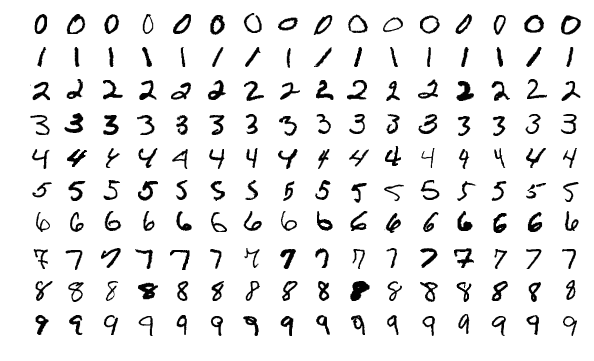MNIST digits
By Josef Steppan - Own work, CC BY-SA 4.0, https://commons.wikimedia.org/w/index.php?curid=64810040

The first thing we do is to linearize the data of each image before putting it into the network. We must note that a computer sees everything including images as numbers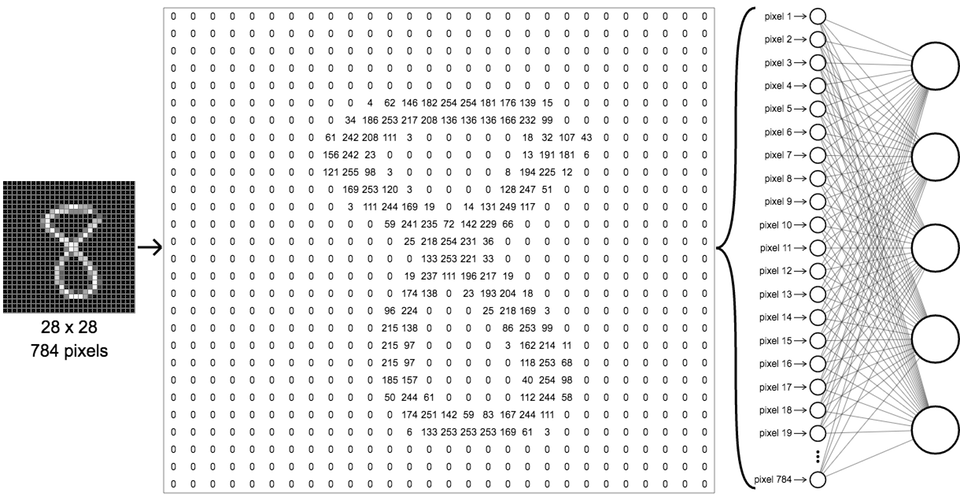Source: http://ml4a.github.io/ml4a/neural_networks/

By linearizing we lay each row of the image pixels values side by side. A typical image of a digit from the MNIST database has the size of 28 by 28 pixels so if we lay it side by side we end up with a vector (list) of 784 pixels. It is the vector of pixels that we input into the neural network.

The whole process of training a neural network is only half of the equation. To use the network we perform a process of inference by querying the network with new data it has not seen before. In our handwriting example, this data will actually be some handwritten digit that we do not have in the database, probably something we or some other person wrote down. We take this image and query the network with it and if it comes up with a good answer we know that we have succeeded in training and deploying a neural network capable of recognizing an image of some class.

There are metrics that help us understand how well our network is performing when tested with some data it has not seen and these are different from the process we do during the training of the network. We evaluate the network to find how many unseen examples it classifies as True positives or True Negatives. A true positive is a cat that is identified as a cat and a true negative is a dog that was not classified as a cat. This gives us an idea of how the network is performing. But to really get to the bottom of it we must also know how many false positives, that is images of dogs that were classified as cats and how many false negatives that are images of cats that were not identified as cats.

These evaluations are done at the inference stage of the neural network design and not during training. During the training of the neural network, the kind of evaluation we do is between the performance on the training set of the data and the test set.

Remember we talked about how we train the network with a single example, where we pass on input, perform the forward propagation check the error between training result and the ideal result and then backpropagate that error.

In practice we do not deal with single examples, rather we deal with a batch of training examples at once. During practical training, we usually divide that data set we are presented with into 3. We, first of all, set out about 70% for training data and 30% for validation and test data. Some people break it up into 80% of the data for training and 20% for validation and test.

The 30% is broken into 15% validation and 15% test data. During the training of the 70% data, we use our 15% validation data to monitor our progress on training. So we train the network and monitor the loss or error and monitor how the network is doing so far on the validation data. The training loss is different from the validation loss, during training we are doing the basic backpropagation and comparing the error with the idea values, while we use the validation data to check the performance of the model so far on data it has not seen.

The main difference between validation and test data is that the validation is like the test data during training, while the main test data is the real test data after training. The validation data enable us to monitor several things that are going on during the training on the network and gives us certain kinds of actionable information.

The 3 critical pieces of information we get from the validation data is whether the network model is overfitting, underfitting or just at the right capacity. If the training loss is going down but the validation loss is going up, then our network is overfitting to the training data. If the training loss is not going down at all then it is underfitting. Underfitting means the network model is not sufficient for learning good features.

If both training and validation loss is going down together then the network is probably at the right capacity and is actually learning. Our goal will then be to reduce the difference between training loss and validation loss.

For simple vanilla handwritten digit classification, a simple deep architecture is all 2 or 3 layers is enough to learn all the classes of the digits. But when it comes to images of dogs and cats, etc. we have to go a little bit further by adding extra layers at the beginning of the network called convolutional layers.

A convolutional layer uses a filter which is a 2-dimensional grid of numbers to perform computation on our input. We have seen that our basic neural network just takes inputs and weights, multiplies them, sums them and applies an activation function. Ignoring the details of these operations we can see that an artificial neuron simply performs some computation on its input and returns the result of that computation.

Convolutional units also perform computation and return output. In order to gain high-level insight, we will summarize the operation of convolutional units as applying several filters to data which prepares the data for being input into fully connected layers, the kind we are used to in regular DNNs.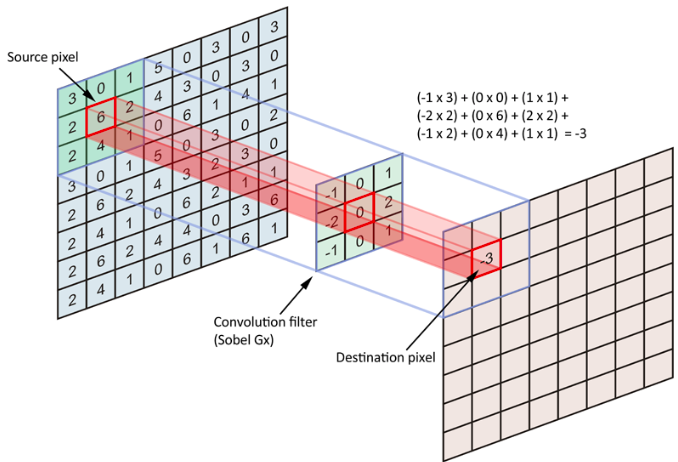https://medium.freecodecamp.org/an-intuitive-guide-to-convolutional-neural-networks-260c2de0a050

The image above is an example showing the application of a convolution filter to a section of the pixel values of an image, although in this particular image the pixel values are not representative of any particular image we can view it as a generalization of pixel values that make up an image. Convolution filters produce what are known as feature maps of their inputs and of course, they can be stacked to produce deep convolution layers.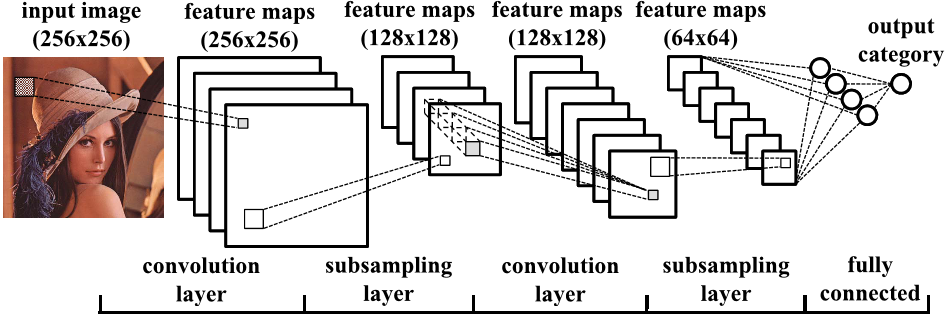Above is a typical architecture for image classification. The convolution layers convolve their input into feature maps and the feature maps are subsampled to reduce their size so as to reduce the number of parameters the machine has to deal with thus improving efficiency.

So far, we have focused on classification which is the easiest use case of deep learning to understand. Another major use case is in regression which involves predicting a single real number or a vector of real numbers.

If you wanted to build a model that predicts housing prices in a particular state given input features like crime rate, median income, number of rooms and even GPS location, etc. and you had target housing prices, then you would be facing a regression problem.

The setup is similar to the classification problem that we dealt with above, the only difference is that rather than coming up with a vector of probabilities with the probability that the input is of a particular class being the highest, you would rather be predicting a single value which represents the target you seek.

The structure of the network would be different and you might not need convolution layers but the network is still a deep network and extracts features from data in the forward step and reduces errors in the backward step till it converges at some fairly correct prediction.

The other side of the intelligence equation after the pattern recognition phase which we currently perform with things like deep learning is the action generation phase. The action generation phase is what makes us look like we are acting with intelligence. Actions can be anything from writing a book, moving towards a favoured member of the opposite sex, inventing a constraint satisfaction problem solver or designing electronic circuitry, etc.

With modern artificial intelligence, we have been able to come up with primitive abstract systems that handle this action generative phase stuff like recurrent neural networks, Deep Reinforcement learning systems, Generative adversarial network systems and some hand-coded advanced algorithms that come from the GOFAI era that do not use data-driven machine learning approach.

The distinguishing feature of modern AI research is that the system improves as more data is made available. In the past GOFAI era progress was driven forward by human cleverness in the invention of new algorithms that outperform previous ones. But in this modern era, systems will generally improve as more data is made available to them. It is hard to know for sure how long data will keep giving us constant improvements but I think we might be approaching an era where we will have to look back into cleverness in algorithm design to make further progress towards our goal of building synthetic intelligence.

At least it is ridiculous that AI systems need hideously large amounts of data to perform simple tasks when humans need just a little amount. Most of the modern image recognition systems start reaching human performance levels when they have been trained with billions of examples and with huge amounts of computing power to spare, but it is amazing that a human with just a few examples and 20W of power in their heads can do more than all these goliaths sized systems.

Many influential intellectuals in the technical field are dismissing this weakness in performance and are instead blaming it on the fact that humans have had millions of years of evolution and thus have experienced more data than these modern machines and thus they justify the clamour for more data.

This is a defeatist point of view and rather than worship data, and of course, there is nothing wrong with more data, I think that what we need is more cleverness. We need more cleverness in algorithm design no different from the kind of fervent research we saw in the early days of AI, that is during the 60s and 70s pre-AI winter period.

Retiring into our seat that our current implementation of generalized network algorithms like our modern deep learning systems is enough and advanced already and that they only way to improve performance is to get more data or more hardware is a weak point of view. There might be more going on in the brain that we might not be aware of and maybe future research might reveal something better than multiply-sum-activate-backpropagate.

In my opinion research into computational neuroscience will reveal lots of insight, and this is not me sanctioning brain-simulation-equals-to-AGI ideas, but I think that if we really understand the brain at the lowest levels, at least above the levels of basic molecules, we might be inspired to create clever algorithms that reduce data and power costs of AI research just like observing the interconnections of neurons has inspired current research.

1.2.### Next Steps Towards Strong Artificial Intelligence

What is Intelligence? Pathways to Synthetic Intelligence If you follow current AI Research then it will be apparent to you that AI research, the deep learning type has stalled! This does not mean that new areas of application for existing techniques are not appearing but that the fundamentals have been solved and things have become pretty standardized.

### How to become an AI researcher

Artificial Intelligence is all the rage these days. Everyone is getting on the bandwagon but there seems to be a shortage of AI researchers everywhere these days. Although many people are talking about doing AI not many people are actually doing AI research.

### What is Intelligence: Strong AI arrival scenarios

Strong AI arrival scenarios There are several scenarios through which Strong AI can arrive for humanity. Here are some that I find highly probable.